### Introduction to Numerical Analysis: Error

### Accuracy and Precision

Accuracy is the measure of how closely a computed or measured value agrees with the true value. Precision is the measure of how closely individual computed or measured values agree with each other. Figure 1 illustrates the classical example of four different situations of five dart board throws to differentiate between accuracy and precision. In the first situation, the five throws are centered around the Bull’s eye (accurate) and are close to each other (precise). In the second situation, the five throws are centered far from the Bull’s eye (inaccurate) but are close to each other (precise). In the third situation, the five throws are centered around the Bull’s eye (accurate) but are far from each other (imprecise). In the last situation, the five throws are centered far from the Bull’s eye (inaccurate) and they are far from each other (imprecise). In general, if we take the average position of the five throws, then, the distance between the average position and the Bull’s eye position would give a measure of the accuracy. On the other hand, the standard deviation of the position of the five throws gives a measure of how precise (close to each other) the throws are.

##### Degree of Precision

For measurement or computational systems, the degree of precision defines the smallest value that can be measured or computed. For example, the smallest division on a scientific measurement device would give the degree of precision of that device. The smallest or largest number that a computer can store defines the degree of precision of a computation by that computer.
For example, Figure 2 shows a ruler with a 1mm degree of precision. To properly record the measurement, the degree of precision has to be indicated. The measurement on the left should be recorded as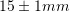. The measurement on the right should be recorded as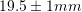.

The degree of precision of a computational device depends on the algorithm of computation and storage capacity of the device. For example, a calculator might do calculations up to a particular accuracy, say ten decimal digits. Traditionally computer coding had different degrees of numerical precision for example: Single precision and double precision. The storage of numbers in these cases uses the “floating point” storage in which a number is stored as a significand and an exponent. More recently, some coding software adopt an arbitrary precision where the number of digits of precision of a number is limited only by the available memory of the computer system. It should be noted that modern computers have the ability to store enough numbers to ensure very high degree of precision for the majority of practical applications, in particular those applications described in these pages.

For the pages here we will be using Mathematica software which uses its own definitions of precision and accuracy. Numerical precision in Mathematica is defined as the number of significant decimal digits while accuracy is defined as the number of significant decimal digits to the right of the decimal point. See the Mathematica page on numerical precision for more details. As an example, consider the number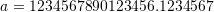. Whenis assigned this number, it automatically adopts its precision which is 23 (23 significant digits). We can then use the command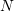to set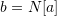. This command stores a rounded value ofwith machine precision (16 significant digits) into b. Therefore,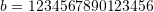. If we subtract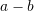, Mathematica uses the least precision in the calculations (machine precision) and so the result is zero. We can reset the precision ofto be 23 significant digits. Then, in that case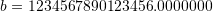. Then, when we subtract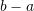we get -0.1234567.
View Mathematica Code

a = 1234567890123456.1234567
b = N[a]
Precision[a]
Precision[b]
b = SetPrecision[b, 23]
b - a


### Random and Systematic Errors

Using the same classical example, we can also differentiate the errors in hitting the target (i.e., the difference between the positions of the dart throws and the Bull’s eye) into two types of error: random error and systematic error. A random error is the error due to natural fluctuations in measurement or computational systems. By definition, these fluctuations are random and therefore, the average random error is zero. For the accurate throws shown in Figure 1 (left column), whether the measurements are precise or not, the average position of the five throws is the Bull’s eye. In other words, the average error is zero.
A systematic error is a repeated “bias” in the measurement or computational system. For the inaccurate throws shown in Figure 1 (right column), the thrower has a tendency or bias to throw into the left bottom side of the Bull’s eye. So, in addition to the random error, there is a systematic error (bias).

An example of a systematic error is if a scale reads 1kg when there is nothing on it so it adds 1 kg to its actual measurement. This additional 1kg is a systematic error.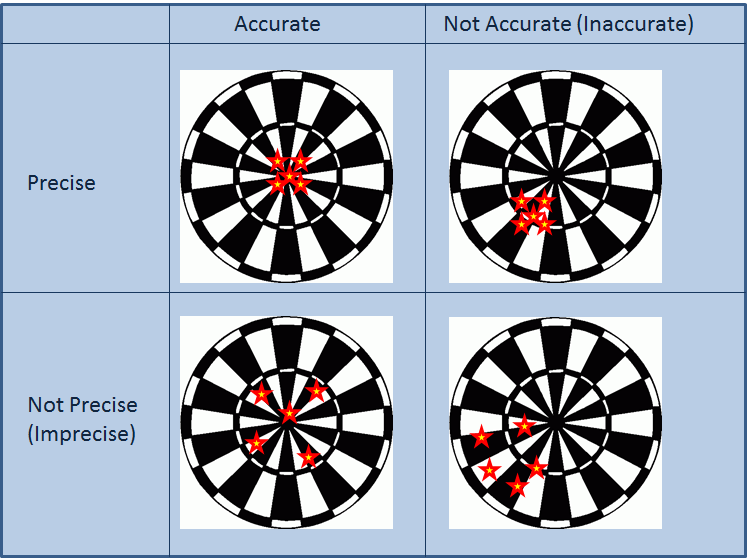Figure 1. Illustration of accuracy and precision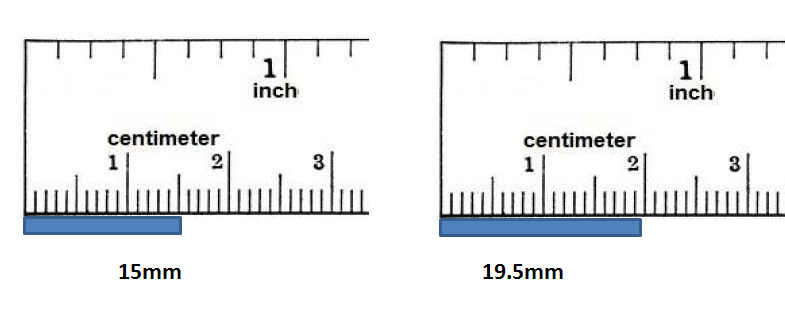Figure 2. Degree of precision of a ruler

### Basic Definitions of Errors

Measurement devices are used to find a measurement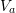as close as possible to the true value. Similarly, numerical methods often seek to find an approximation for the true solution of a problem. The error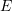in a measurement or in a solution of a problem is defined as the difference between the true valueand the approximation.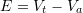Another measure is the relative error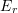. The relative error is defined as the value of the errornormalized to the true value: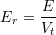In general, if we don’t know the true value, there are methods for estimating an approximation for the error. Ifis an approximation for the error, then, the relative approximate error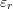is defined as: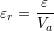##### Word of Caution

It is important to note that for a particular computation, if the true value is equal to zero, then the relative measures of error need to be viewed carefully. In particular, in a numerical procedure, when the true value is not known, the relative error is a quantity that is approachingwhich might not show any sign of convergence. In these situations, it is advisable to have other measures of convergence such as the value of the difference of the estimates or the value ofitself.

### Errors in Computations

There are two types of errors that arise in computations: round-off errors and truncation errors.

#### Round-off Errors

Round-off error is the difference between the rounding approximation of a number and its exact value. For example, in order to use the irrational numberin a computation, an approximate value is used. The difference betweenand the approximation represents the rounding error. For example, using 3.14 as an approximation forhas a rounding approximate error of around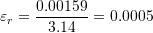When we use a computational device, numbers are represented in a decimal system. The precision of the computation can be defined using different ways. One way is to define the precision up to a specified decimal place. For example, the irrational numberapproximated to the nearest 5 decimal places is 3.14159. In this case, we know that the error is less than 0.00001. Another way is to define the precision up to a specified number of significant digits. For example, the irrational numberapproximated to 5 significant digits is 3.1416. Similarly, we know that the error in that case is less than 0.0001.

Round-off errors have an effect when a computation is done in multiple steps where a user is rounding off the values rather than using all the digits stored in the computer. For example, if you divide 1 by 3 in a computer, you get 0.333333333333. If you then round it to the nearest 2 decimal places, you get the number 0.33. If afterwards, you multiply 0.33 by 3, you get the value 0.99 which should have been 1 if rounding had not been used.

Even when computers are used, in very rare situations, round-off errors could lead to accumulation of errors. For example, rounding errors would be significant if we try to divide a very large number by a very small number or the other way around. Another example is if a calculation involves numerous steps and rounding is performed after every step. In these situations, the precision and/or rounding by the software or code used should be carefully examined. Some famous examples of round-off errors leading to disasters can be found here. See example 4 below for an illustration of the effect of round-off errors.

#### Truncation Errors

Truncation errors are errors arising when truncating an infinite sum and approximating it by a finite sum. Truncation errors arise naturally when using the Taylor series, numerical integration, and numerical differentiation. These will be covered later along with their associated truncation errors. For example, as will be shown later, the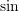function can be represented using the infinite sum: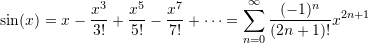In fact, this is how a calculator calculates the sine of an angle. Using the above series,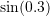where 0.3 is in radian can be calculated using the first two terms as: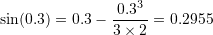which in fact is a very good approximation to the actual value which is around 0.295520207. The error, or the difference between 0.2955 and 0.295520207 is called a truncation error arising from using a finite number of terms (in this case only 2) in the infinite series.

##### Error Estimation in Computational Iterative Methods

If the approximate valueis obtained using an iterative method, i.e., each iterationwould produce the approximation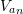, then, the relative approximate error is defined as: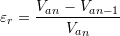In such cases, the iterative method can be stopped when the absolute value of the relative error reaches a specified error level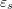: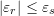### Examples and Problems

#### Example 1

The width of a concrete beam is 250mm and the length is 10m. A length measurement device measured the width at 247mm while the length was measured at 9,997mm. Find the error and the relative error in each case.

##### Solution

For the width of the beam, the error is: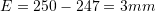The relative error is: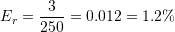For the length of the beam, the error is: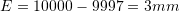The relative error is: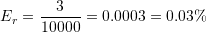This example illustrates that the value of the error itself might not give a good indication of how accurate the measurement is. The relative error is a better measure as it is normalized with respect to the actual value. It should be noted however, that sometimes the relative error is not a very good measure of accuracy. For example, if the true value is very close to zero, then the relative error might be a number that is approximately equal toand so it can give very unpredictable values. In that case, the errormight be a better measure of error.

#### Example 2

Use the Babylonian method to find the square roots of 23.67 and 19532. For each case use an initial estimate of 1. Use a stopping criterion of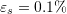. Report the answer

1. to 4 significant digits.
2. to 4 decimal places.
##### Solution

The following table shows the Babylonian-method iterations to find the square root of 23.67 until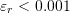. Excel was used to produce the table. The criterion for stoppage was achieved after 6 iterations.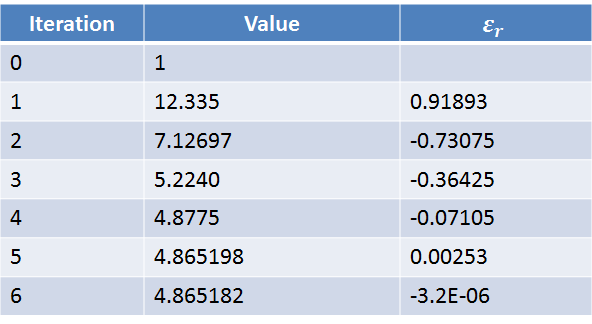Therefore, the square root of 23.67 to 4 significant digits is 4.865. The square root of 23.67 to 4 decimal places is 4.8652.

The following table shows the Babylonian-method iterations to find the square root of 19532 until. Excel was used to produce the table. The criterion for stoppage was achieved after 11 iterations.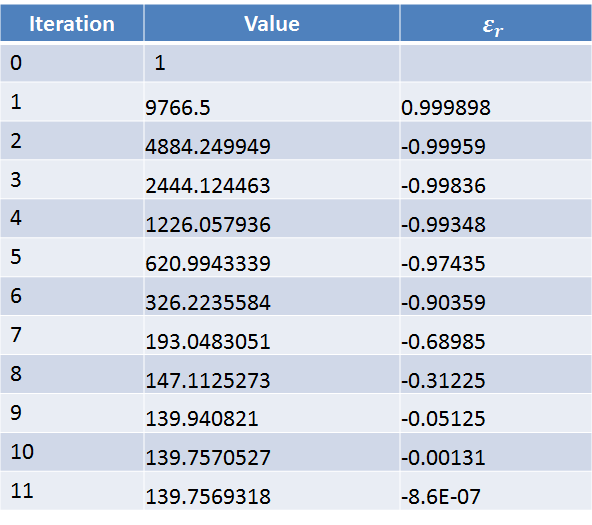Therefore, the square root of 19532 to 4 significant digits is 139.8. The square root of 19532 to 4 decimal places is 139.7569.

You can use Mathematica to show the square root of these numbers up to as many digits as you want. Notice that we used a fraction to represent 23.67, otherwise, it would resort to machine precision which could be less than the number of digits specified. Copy and paste the following code into your Mathematica to see the results:
View Mathematica Code:

t1=N[Sqrt[2367/100],200]
t3 = N[Sqrt, 200]


The Babylonian method to find the square root of a number can also be coded in Matlab. You can download the Matlab file below:

#### Example 3

The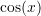function whereis in radian can be represented as the infinite series: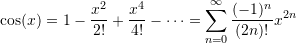Letbe the number of terms used to approximate the function. Draw the curve showing the relative approximate error as a function of the number of terms used when approximating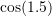and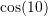. Also, draw the curve showing the value of the approximation in each case as a function of.

##### Solution

We are going to use the Mathematica software to produce the plot. First, a function will be created that is a function of the value ofand the number of terms. This function has the form: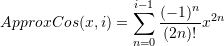For example,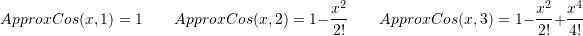The relative approximate error when usingterms can be calculated as follows: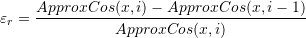A table of the relative error forbetween 2 and 20 can then be generated. Finally, the list of relative errors and the approximations can be plotted using Mathematica.
Note the following in the code. The best way to achieve good accuracy is to define the approximation function with arbitrary precision. Therefore, no decimal points should be used. Otherwise, machine precision would be used and might lead to deviation from the accurate solution as will be shown in the next example. The angles are defined using fractions and after evaluating the functions, the numerical evaluation function N[] is used to numerically evaluate the approximate value after adding enough terms!
View Mathematica Code

ApproxCos[x_, i_] := Sum[(-1)^n*x^(2 n)/((2 n)!), {n, 0, i - 1}]
Angle1 = 15/10;
Angle2 = 10;
ertable1 = Table[{i, N[(ApproxCos[Angle1, i] - ApproxCos[Angle1, i - 1])/ApproxCos[Angle1, i]]}, {i, 2, 20}]
ertable2 = Table[{i, N[(ApproxCos[Angle2, i] - ApproxCos[Angle2, i - 1])/ApproxCos[Angle2, i]]}, {i, 2, 20}]
ListPlot[{ertable1, ertable2}, PlotRange -> All, Joined -> True, AxesLabel -> {"i", "relative error"}, AxesOrigin -> {0, 0}, PlotLegends -> {"Relative error in Cos(1.5)", "Relative error in Cos(10)"}]
cos1table = Table[{i, N[ApproxCos[Angle1, i]]}, {i, 2, 20}]
cos2table = Table[{i, N[ApproxCos[Angle2, i]]}, {i, 2, 20}]
ListPlot[{cos1table, cos2table}, Joined -> True,  AxesLabel -> {"i", "Cos approximation"}, AxesOrigin -> {0, 0},  PlotLegends -> {"Cos(1.5) approximation", "Cos(10) approximation"}, PlotRange -> {{0, 20}, {-10, 10}}]
N[ApproxCos[Angle1, 20]]
N[ApproxCos[Angle2, 20]]


Approximate the function cos(x) with a specified number of terms is coded in Matlab as follows. The main script calls the function approxcos prepared in another file and outputs the approximation.

The plot of the relative error is shown below. For, the relative error decreases dramatically and is already very close to zero when 4 terms are used! However, for, the relative error starts approaching zero when 14 terms are used!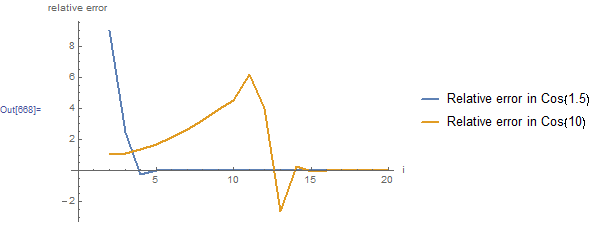The plot of the approximate values ofandas a function of the number of terms is shown below. The approximation forapproaches its stabilized and accurate value of 0.0707372 using only 3 terms. However, the approximation forkeeps oscillating taking very high positive values followed by very high negative values as a function of. The approximation starts approaching its stabilized and accurate value of around -0.83907 after using 14 terms!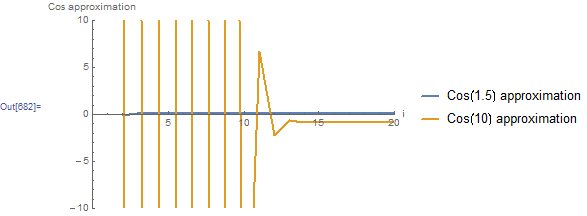#### Example 4

Thefunction whereis in radian can be represented as the infinite series:Letbe the number of terms used to approximate the function. Show the difference between using machine precision and arbitrary precision in Mathematica in the evaluation of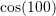.

##### Solution

Each positive term in the infinite series ofis followed by a negative term. In addition, each term has a value of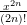. For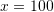, the series would be composed of adding and subtracting very large numbers. If machine precision is used, then round-off errors will lead to the inability of the series to actually converge to the correct solution. The code below shows that using 140 terms, the series gives the value of 0.862314 which is a very accurate representation of. This value is obtained by first evaluating the sum using arbitrary precision and then numerically evaluating the result using machine precision. If, on the other hand, we use a machine precision for the angle, the computations are done in machine precision. In this case, the series converges, however, to the value of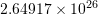! This is because in effect we are adding and subtracting very large numbers, each with a small round-off error because of using machine precision. This problem is termed Loss of Significance. Since the true value is much smaller than the individual terms, then, the accuracy of the calculation relies on the accuracy of the differences between the terms. If only a certain number of significant digits is retained, then, subtracting each two large terms leads to loss of significance!

View Mathematica Code
ApproxCos[x_, i_] := Sum[(-1)^n*x^(2 n)/((2 n)!), {n, 0, i - 1}]
N[ApproxCos[100, 140]]
ApproxCos[N, 140]
ApproxCos[N, 300]
Cos[100.]


#### Problems

1. Use the Babylonian method to find the square root of your ID number. Use an initial estimate of 1. Use a stopping criterion of. Report the answer in two formats: 1) approximated to 4 significant digits, and 2) to 4 decimal places.
2. Find two examples of random errors and two examples of systematic errors.
3. Find a calculating device (your cell phone calculator) and describe what happens when you divide 1 by 3 and then multiply the result by 3. Repeat the calculation by dividing 1 by 3, then divide the result by 7. Then, take the result and multiply it by 3, then multiply it by 7. Can you infer how your cell phone calculator stores numbers?
4. Using the infinite series representation of thefunction to approximate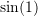and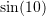, draw the curve showing the relative approximate error as a function of the number of terms used for each.
5. Using Machine Precision in Mathematica, find a numberthat satisfies: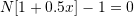while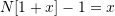. Compare that number with MachineEpsilon in Mathematica

.